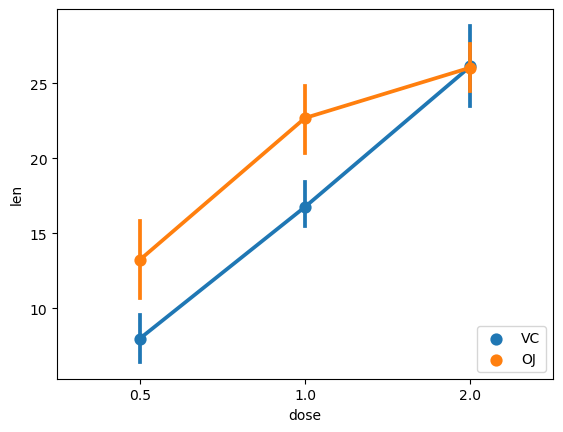# How to plot interaction effects of treatments (in Python, using Matplotlib and Seaborn)

See all solutions.

When there are multiple treatment conditions with multiple levels and you wish to undertsand the interaction effects of each of them, a plot can be useful. How can we create the right kind of plot for that situation?

## Solution

The solution below uses an example dataset about the teeth of 10 guinea pigs at three Vitamin C dosage levels (in mg) with two delivery methods (orange juice vs. ascorbic acid). (See how to quickly load some sample data.)

1
2
from rdatasets import data
df = data('ToothGrowth')


To plot the interaction effects among tooth length, supplement, and dosage, we can use the pointplot function in the Seaborn package.

1
2
3
4
5
import seaborn as sns
import matplotlib.pyplot as plt
sns.pointplot(x='dose',y='len',hue='supp',data=df)
plt.legend(loc='lower right')  # Default is upper right, which overlaps the data here.
plt.show()Looking at the output, we first see that there is an interaction effect because the two supp lines intersect. We also see that there is a difference in length when giving 0.5mg and 1mg dosage of either of the two delivery methods. However, there is barely any difference between the delivery methods when the dosage level is 2mg.